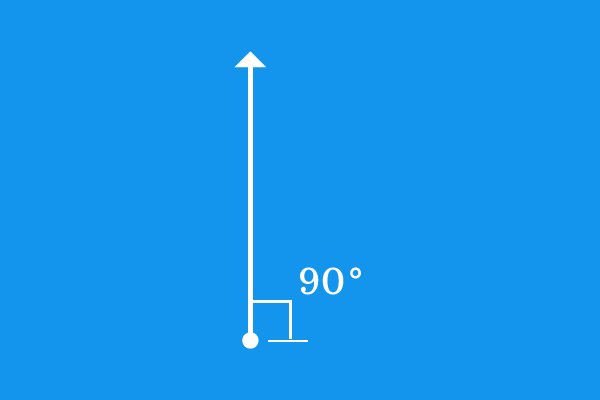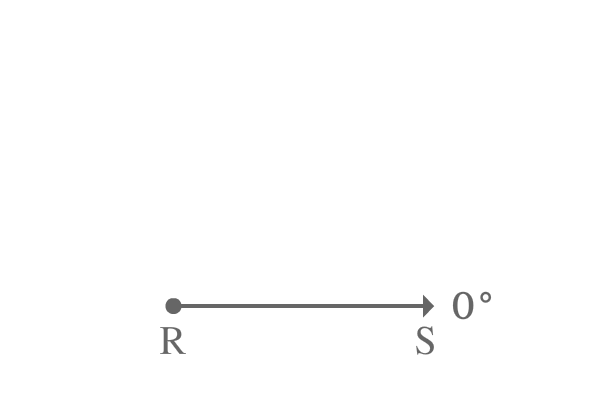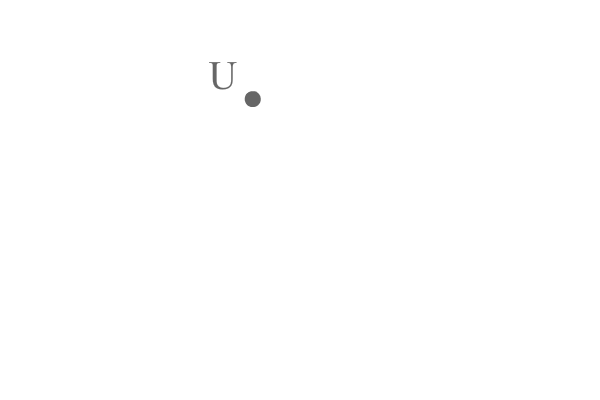# Right angleAn angle of $90^\circ$ is called a right angle.

The meaning of right angle is $90^\circ$, if any angle is $90^\circ$, the angle is called a right angle.

Right angle is denoted by a symbol $∟$ in mathematics.

## Representation

A right angle is usually written in three ways in mathematics.

$(1) \,\,\,\,\,\,$ $90^°$ (in Degrees).

$(2) \,\,\,\,\,\,$ $\dfrac{\pi}{2}$ (in Radians)

$(3) \,\,\,\,\,\,$ $100^g$ (in Gradians)

### Examples

Right angle is formed in two different types in geometric system.

#### Right angle by a Line

Any angle of a line is generally measured from zero degrees line. If any line is exactly $90^\circ$ to the surface of the earth, the angle made by the line is called a right angle.$\overrightarrow{RS}$ is a ray and it is initially parallel to the surface of the earth. In other words, it is at zero angle.

The ray $\overrightarrow{RS}$ is rotated an angle of $90^\circ$ in anticlockwise direction to reach its final position where the ray $\overrightarrow{RS}$ is known as $\overrightarrow{RT}$.

The angle made by the ray $\overrightarrow{RS}$ from its initial position to final position is $90^\circ$ and it is denoted by $\angle TRS$.

$\angle TRS = 90^\circ$

The angle $TRS$ is exactly $90^\circ$. Therefore, the angle is called a right angle.

#### Right angle between two lines

The angle between any two straight lines is measured geometrically by considering one line as baseline to another line and vice-versa to measure the angle. If the angle between them is $90^\circ$, the two lines are perpendicular to each other and the angle made between them is called a right angle.$\overrightarrow{UV}$ and $\overrightarrow{UW}$ are two rays and they both are started from a point $U$ but they continued travelling perpendicular to each other.

So, the angle between $\overrightarrow{UV}$ and $\overrightarrow{UW}$ is $90^\circ$ and the angle between them is denoted by $\angle VUW$.

$\angle VUW = 90^\circ$

The angle $VUW$ is $90^\circ$, therefore the angle between rays $\overrightarrow{UV}$ and $\overrightarrow{UW}$ is called a right angle.

A best free mathematics education website that helps students, teachers and researchers.

###### Maths Topics

Learn each topic of the mathematics easily with understandable proofs and visual animation graphics.

###### Maths Problems

A math help place with list of solved problems with answers and worksheets on every concept for your practice.

Learn solutions

###### Subscribe us

You can get the latest updates from us by following to our official page of Math Doubts in one of your favourite social media sites.